# KSEEB Solutions for Class 6 Maths Chapter 13 Symmetry Ex 13.2

Students can Download Chapter 13 Symmetry Ex 13.2 Questions and Answers, Notes Pdf, KSEEB Solutions for Class 6 Maths helps you to revise the complete Karnataka State Board Syllabus and score more marks in your examinations.

## Karnataka State Syllabus Class 6 Maths Chapter 13 Symmetry Ex 13.2

Question 1.
Find the number of lines of symmetry for each of the following shapes;Solution :
a) There are 4 lines of symmetry for the given figure
b) There are 4 lines of symmetry for the given figure
c) There are 4 lines of symmetry for the given figure
d) There is only 1 line of symmetry for the given figure
e) There are 6 lines of symmetry for the give figure.
f) There are 6 lines of symmetry for the given figure
g) There is no line of symmetry for the given figure
h) There is no line of symmetry for the given figure.
i) There are 3 lines of symmetry for the given figure.
The required triangle can be formed as follows

Question 2.
Copy the triangle in each of the following figure on squared paper In each case, draw the line (s) of symmetry if any and identify the type of (some of you may like to trace the figures and try paper – folding first!).Solution:
a) It is an isosceles ∆le. There will be only 1 line of symmetryb) It is an isosceles triangle There will be only 1 line of Symmetry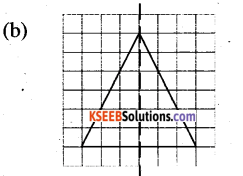c) It is a right triangle. There will be only 1 line of symmetryd) It is a scalene ∆le There will be no line of symmetryQuestion 3.
Complete the following table.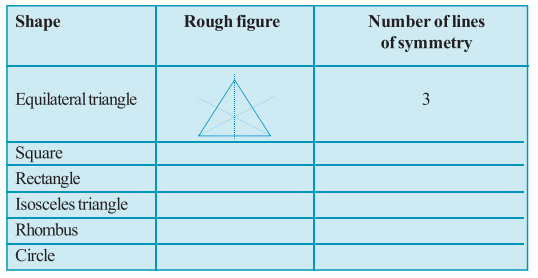Solution:In case of a circle, there are infinite lines In the above table, only some line symmetry are drawn more symmetric lines can be similarly drawn for it.

Question 4.
Can you draw a triangle which has
Solution:
a) Exactly one line of symmetry?
Yes, we can make an isosceles ∆ le Which has 1 line of symmetry.b) Exactly two line of symmetry?
No, we cannot draw such a triangle

c) Exactly three lines of symmetry?
Yes, we can make an equilateral ∆ le Which has 3 lines of symmetry.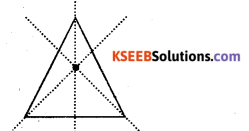d) No lines of symmetry?
Yes, we can make a scalene ∆ le Which has no line of symmetry.Question 5.
On a squared paper, sketch the following:
Solution:
a) A triangle with a horizontal line of symmetry but no vertical line of symmetry.
A triangle with only I horizontal line of symmetry and no other vertical line of
symmetry can be sketched as follows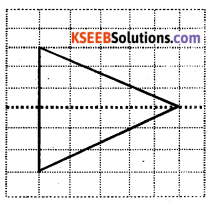b) A quadrilateral with both horizontal and vertical lines of symmetry.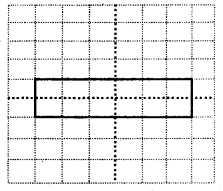c) A quadrilateral with a horizontal line of symmetry but no vertical line of symmetry.d) A hexagon with exactly two lines of symmetry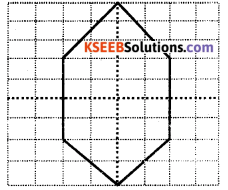e) A hexagon with six lines of symmetry.(Hint: It will be helpful if you first draw the lines of symmetry and then complete the figures)Question 6.
Trace each figure and draw the lines of symmetry, if any: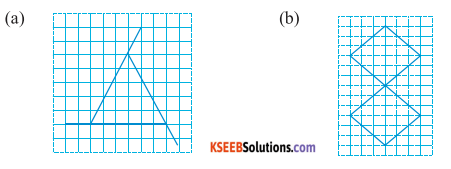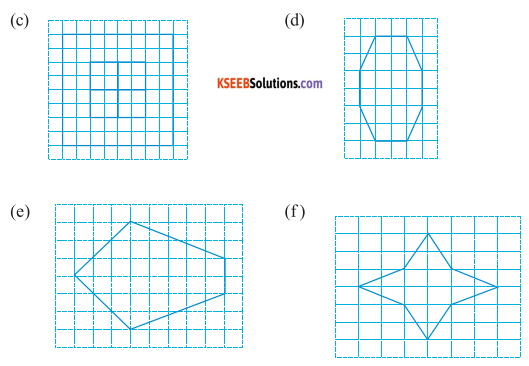Solution: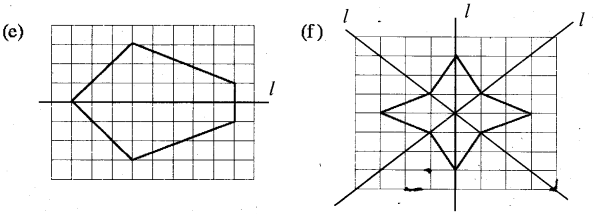a) The given figure is an isosceles ∆ le Therefore, these will be 2 line of symmetry.
b) The given figure has 2 lines of symmetry.
c) The given figure has 4 lines of symmetry
d) The given figure is an octagonal having 2 lines of symmetry
e) The given figure has only 1 line of symmetry.
f) The given figure has 4 lines of symmetry .

Question 7.
Consider the letters of English alphabets, A to Z . List among them the letters Which have
Solution:a) Vertical lines of symmetry (like A)
A, H, I, M, 0, T, U, V, W, X, Y

b) Horizontal lines of symmetry (like B)
B, C, D, E, H, I, K, Q, X

c) No lines of symmetry (like Q)
F, G, J, L, N, P, Q, R, S, ZQuestion 8.
Given here are figures of a few folded sheets and designs drawn about the fold. In each case, draw a rough diagram of the complete figure that would be seen when the design is cut off.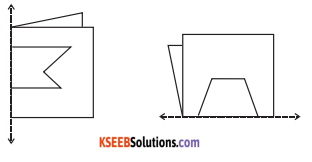Solution: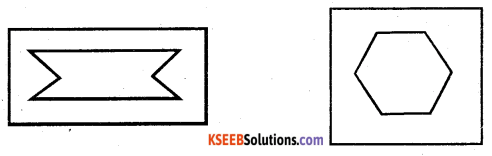error: Content is protected !!